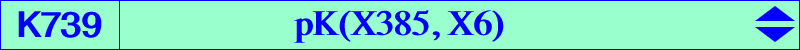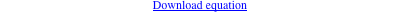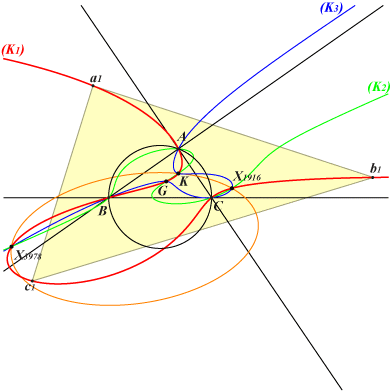∑ (a^4 - b^2c^2)(c^2 y - b^2 z) y z = 0X(2), X(6), X(83), X(194), X(239), X(287), X(385), X(732), X(894), X(1916), X(3225), X(3978) infinite points of the Steiner ellipse A'B'C' cevian triangle of X(6)The first anti-Brocard triangle and the anticevian triangle of M are perspective if and only if M lies on K739. The locus of the perspector is K699. See the related K738. See also CL041. The isogonal transform of K739 is K787 = pK(X9468, X9468) and its isotomic transform is K322. K739 is a cubic anharmonically equivalent to K020 as in Table 66. The symbolic substitution SS{a -> √a} transforms K739 into K770. K738 and K739 generate a pencil of circum-cubics passing through X(2), X(6), X(1916), X(3978) and the infinite points S1, S2 of the Steiner ellipse. This pencil contains a third (and last) pK which is K354.The tangents at S1, S2 to a cubic (K) of the pencil meet at a point F which lies on the ellipse passing through X(6), X(1916), X(3978), S1, S2. F lies on (K) if and only if it is one of these points X(6), X(1916), X(3978) corresponding respectively to three cubics (K2), (K3), (K1) very similar to focal cubics. The most interesting is probably (K1) since it contains the vertices of the first anti-Brocard triangle and X(671), the last common point with the Steiner ellipse. (K2) passes through X(538). (K3) passes through X(524), X(598), X(3228), this latter point on the Steiner ellipse.